# Multiplication Table (Times Table)

Use this multiplication table to learn times tables in seconds. Hover your cursor on table and see the result. For example, if you are looking for 14 comes in which table, locate 14 in this table and you will get the answer below.

i
i
 × 1 2 3 4 5 6 7 8 9 10 11 12 13 14 15 1 1 2 3 4 5 6 7 8 9 10 11 12 13 14 15 2 2 4 6 8 10 12 14 16 18 20 22 24 26 28 30 3 3 6 9 12 15 18 21 24 27 30 33 36 39 42 45 4 4 8 12 16 20 24 28 32 36 40 44 48 52 56 60 5 5 10 15 20 25 30 35 40 45 50 55 60 65 70 75 6 6 12 18 24 30 36 42 48 54 60 66 72 78 84 90 7 7 14 21 28 35 42 49 56 63 70 77 84 91 98 105 8 8 16 24 32 40 48 56 64 72 80 88 96 104 112 120 9 9 18 27 36 45 54 63 72 81 90 99 108 117 126 135 10 10 20 30 40 50 60 70 80 90 100 110 120 130 140 150 11 11 22 33 44 55 66 77 88 99 110 121 132 143 154 165 12 12 24 36 48 60 72 84 96 108 120 132 144 156 168 180 13 13 26 39 52 65 78 91 104 117 130 143 156 169 182 195 14 14 28 42 56 70 84 98 112 126 140 154 168 182 196 210 15 15 30 45 60 75 90 105 120 135 150 165 180 195 210 225

Times Table Worksheets

Give Us Feedback

## Multiplication Table

Memorizing tables is a task that most of the kids don’t like. We have made it easier for students to learn multiplication tables using multiplication chart.

The chart above is printable and can be downloaded with one click.

## What is a multiplication table chart?

The multiplication table chart is that table that displays the multiplication of two numbers. Generally, the multiplication table is written by taking the set of numbers from 1 to 15 at the topmost row and the first left column.

The multiplication chart is a time-saving way during calculations. The multiplication chart of `1 to 15` contains 1-15 numerals in the first row and column.

All the other rows and columns of the `multiplication table` show the product of two numbers one from the first column while the other is from the first row.

Such as, the product of 8 & 6 is 48.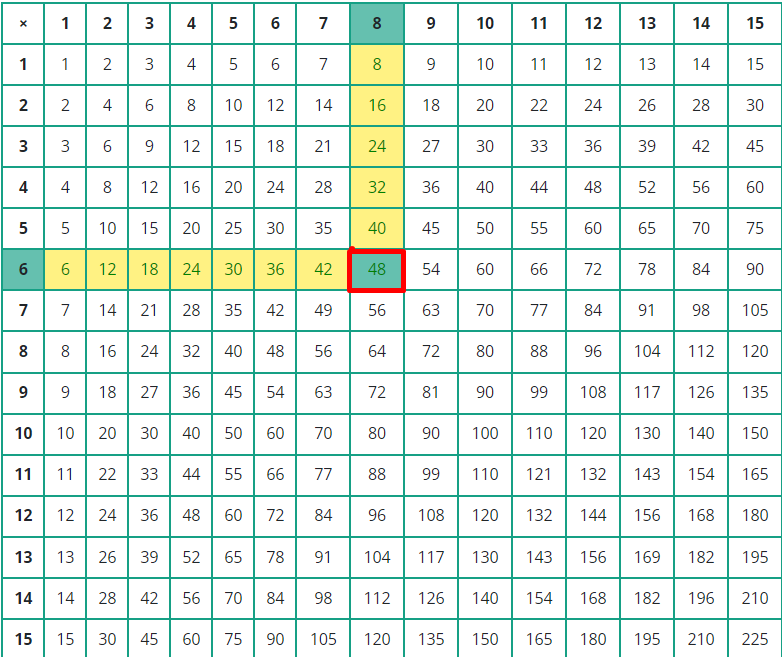Similarly, the product of 13 & 14 is: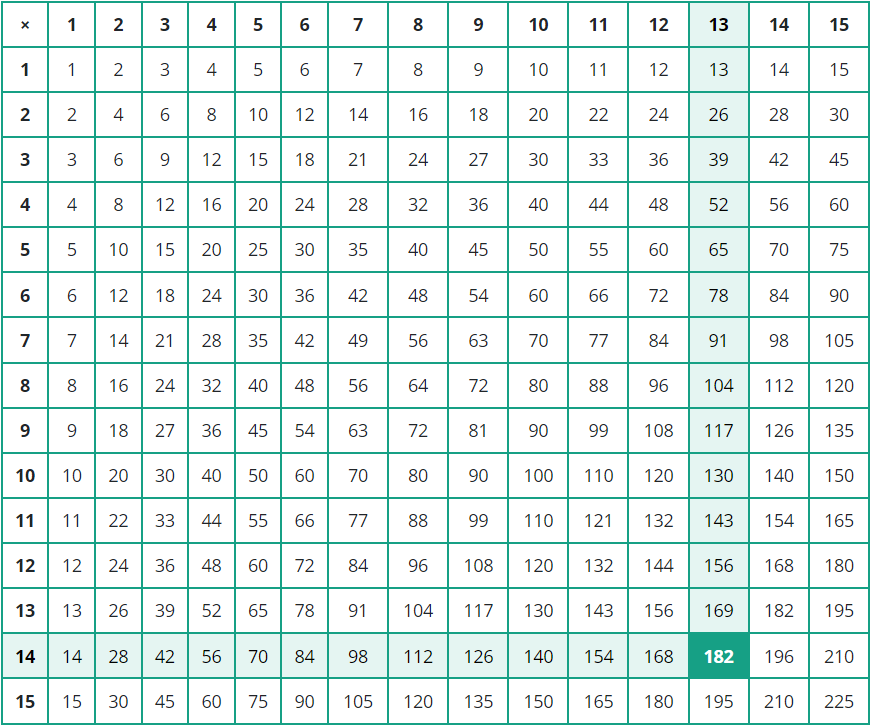## Times Tables 2 to 20

`Multiplication table 2 to 20` are the building blocks of multidigit numbers used to solve the problems of long multiplication, fractions, percentages, and factorization of numbers. The multiplication tables are very helpful for the kids to calculate mental arithmetic problems.

After learning the `times tables from 2 to 20`, kids may become experts in solving complex mathematical problems. The multiplication tables 2 to 20 are given below.

### Times tables 2 to 5

 Times Table of 2 Times Table of 3 Times Table of 4 Times Table of 5 2 x 1 = 2 3 x 1 = 3 4 x 1 = 4 5 x 1 = 5 2 x 2 = 4 3 x 2 = 6 4 x 2 = 8 5 x 2 = 10 2 x 3 = 6 3 x 3 = 9 4 x 3 = 12 5 x 3 = 15 2 x 4 = 8 3 x 4 = 12 4 x 4 = 16 5 x 4 = 20 2 x 5 = 10 3 x 5 = 15 4 x 5 = 20 5 x 5 = 25 2 x 6 = 12 3 x 6 = 18 4 x 6 = 24 5 x 6 = 30 2 x 7 = 14 3 x 7 = 21 4 x 7 = 28 5 x 7 = 35 2 x 8 = 16 3 x 8 = 24 4 x 8 = 32 5 x 8 = 40 2 x 9 = 18 3 x 9 = 27 4 x 9 = 36 5 x 9 = 45 2 x 10 = 20 3 x 10 = 30 4 x 10 = 40 5 x 10 = 50 2 x 11 = 22 3 x 11 = 33 4 x 11 = 44 5 x 11 = 55 2 x 12 = 24 3 x 12 = 36 4 x 12 = 48 5 x 12 = 60

### Times tables 6 to 9

 Times Table of 6 Times Table of 7 Times Table of 8 Times Table of 9 6 x 1 = 6 7 x 1 = 7 8 x 1 = 8 9 x 1 = 9 6 x 2 = 12 7 x 2 = 14 8 x 2 = 16 9 x 2 = 18 6 x 3 = 18 7 x 3 = 21 8 x 3 = 24 9 x 3 = 27 6 x 4 = 24 7 x 4 = 28 8 x 4 = 32 9 x 4 = 36 6 x 5 = 30 7 x 5 = 35 8 x 5 = 40 9 x 5 = 45 6 x 6 = 36 7 x 6 = 42 8 x 6 = 48 9 x 6 = 54 6 x 7 = 42 7 x 7 = 49 8 x 7 = 56 9 x 7 = 63 6 x 8 = 48 7 x 8 = 56 8 x 8 = 64 9 x 8 = 72 6 x 9 = 54 7 x 9 = 63 8 x 9 = 72 9 x 9 = 81 6 x 10 = 60 7 x 10 = 70 8 x 10 = 80 9 x 10 = 90 6 x 11 = 66 7 x 11 = 77 8 x 11 = 88 9 x 11 = 99 6 x 12 = 72 7 x 12 = 84 8 x 12 = 96 9 x 12 = 108

### Times tables 10 to 13

 Times Table of 10 Times Table of 11 Times Table of 12 Times Table of 13 10 x 1 = 10 11 x 1 = 11 12 x 1 = 12 13 x 1 = 13 10 x 2 = 20 11 x 2 = 22 12 x 2 = 24 13 x 2 = 26 10 x 3 = 30 11 x 3 = 33 12 x 3 = 36 13 x 3 = 39 10 x 4 = 40 11 x 4 = 44 12 x 4 = 48 13 x 4 = 52 10 x 5 = 50 11 x 5 = 55 12 x 5 = 60 13 x 5 = 65 10 x 6 = 60 11 x 6 = 66 12 x 6 = 72 13 x 6 = 78 10 x 7 = 70 11 x 7 = 77 12 x 7 = 84 13 x 7 = 91 10 x 8 = 80 11 x 8 = 88 12 x 8 = 96 13 x 8 = 104 10 x 9 = 90 11 x 9 = 99 12 x 9 = 108 13 x 9 = 117 10 x 10 = 100 11 x 10 = 110 12 x 10 = 120 13 x 10 = 130 10 x 11 = 110 11 x 11 = 121 12 x 11 = 132 13 x 11 = 143 10 x 12 = 120 11 x 12 = 132 12 x 12 = 144 13 x 12 = 156

### Times tables 14 to 17

 Times Table of 14 Times Table of 15 Times Table of 16 Times Table of 17 14 x 1 = 14 15 x 1 = 15 16 x 1 = 16 17 x 1 = 17 14 x 2 = 28 15 x 2 = 30 16 x 2 = 32 17 x 2 = 34 14 x 3 = 42 15 x 3 = 45 16 x 3 = 48 17 x 3 = 51 14 x 4 = 56 15 x 4 = 60 16 x 4 = 64 17 x 4 = 68 14 x 5 = 70 15 x 5 =75 16 x 5 = 80 17 x 5 = 85 14 x 6 = 84 15 x 6 = 90 16 x 6 = 96 17 x 6 = 102 14 x 7 = 98 15 x 7 = 105 16 x 7 = 112 17 x 7 = 119 14 x 8 = 112 15 x 8 = 120 16 x 8 = 128 17 x 8 = 136 14 x 9 = 126 15 x 9 = 135 16 x 9 = 144 17 x 9 = 153 14 x 10 = 140 15 x 10 = 150 16 x 10 = 160 17 x 10 = 170 14 x 11 = 154 15 x 11 = 165 16 x 11 = 176 17 x 11 = 187 14 x 12 = 168 15 x 12 = 180 16 x 12 = 192 17 x 12 = 204

### Times tables 18 to 20

 Times Table of 18 Times Table of 19 Times Table of 20 18 x 1 = 18 19 x 1 = 19 20 x 1 = 20 18 x 2 = 36 19 x 2 = 38 20 x 2 = 40 18 x 3 = 54 19 x 3 = 57 20 x 3 = 60 18 x 4 = 72 19 x 4 = 76 20 x 4 = 80 18 x 5 = 90 19 x 5 =95 20 x 5 = 100 18 x 6 = 108 19 x 6 = 114 20 x 6 = 120 18 x 7 = 126 19 x 7 = 133 20 x 7 = 140 18 x 8 = 144 19 x 8 = 152 20 x 8 = 160 18 x 9 = 162 19 x 9 = 171 20 x 9 = 180 18 x 10 = 180 19 x 10 = 190 20 x 10 = 200 18 x 11 = 198 19 x 11 = 209 20 x 11 = 220 18 x 12 = 216 19 x 12 = 228 20 x 12 = 240

## Multiplication table 1-100

To learn and download `multiplication table 1-100`, use the above multiplication table and write any number to generate the table into the box and hit the generate button. The times table will show in a fraction of a second.

You can even generate multiplication tables 1-1000 or more.

## Tips and tricks to learn and memorize the times tables

Kids at the initial stage of their studies are usually struggling to learn and memorize times tables. Below are a few tips and tricks for kids to learn and memorize multiplication tables.

To write and learn any times table start with a natural number and keep adding a similar number in each output. For example, if you want to learn and write the times table of 6, then keep adding 6 in each count. Such as

• 6 x 1 = 6
• 6 x 2 = 6 + 6 = 12
• 6 x 3 = 12 + 6 = 18
• 6 x 4 = 18 + 6 = 24
• 6 x 5 = 24 + 6 = 30
• 6 x 6 = 30 + 6 = 36
• 6 x 7 = 36 + 6 = 42
• 6 x 8 = 42 + 6 = 48
1. ### Keep repeating a table in order

To learn and memorize any table keep repeating the table in order, for example, start learning as

• 4 times 1 is 4
• 4 times 2 is 8
• 4 times 3 is 12
• 4 times 4 is 16
• 4 times 5 is 20

And so on. Keep learning the table again and again until you master it.

1. ### Keep repeating a table backward

To learn the times tables on a fingertip, keep learning and repeating the tables from start to end and vice versa. Scuffle the counting of tables to learn the multiplication tables. Such as,

• 8 times 12 is 96
• 8 times 11 is 88
• 8 times 10 is 80
• 8 times 9 is 72
• 8 times 8 is 64
• And so on.
1. ### Learn and write times tables

Kids could write the times tables daily and recite them daily to memorize the times tables quickly. The learning and writing trick is used when kids feel difficulty memorizing the times tables.

1. ### Use multiplication in daily life

To learn and understand the times tables more accurately, try to apply multiplication in daily life. For example, buy different items such as chips, toffee, and other similar terms. Then multiply the price of one item with the number of items by increasing any item one by one.

1. ### Identify the pattern

Each times table has a pattern. Try to identify and learn the pattern of each times table. It’ll be very helpful in memorizing the times tables faster.

## What tables you should learn first?

You should learn 1, 2, 5, and 10 times table first. These tables are easy as compared to the rest of them. After memorizing these tables, you should memorize 3, 4, 6, 7, 8, and 9 times table.

The most favorite tables of kids are 10 and 11 times tables because it is fun to memorize both of them.

## How to quickly learn times tables?

Here are a few tips to memorize tables quickly.

• Use the times table chart consistently.
• Start learning from the easiest ones.
• Learn addition and subtraction before memorizing times tables.
• Practice multiplication facts (tables) on a daily basis.
• Practice recalling numbers, i.e., in which table 78 comes or 45 comes in which table.

## FAQ

How do you teach multiplication to struggling students?

Students can learn and memorize multiplication with the help of multiplication table charts, flashcards, and other mathematical puzzles.

Why do we use multiplication tables?

A multiplication table is used to find the product of any two numbers and factors of any numbers. For example, the product of 2 & 3, 143 comes in which table, 117 comes in which table, 182 comes in which table, 156 comes in which table, etc.

How to see factors on a multiplication table?

Use a multiplication table chart to view the factors of numbers. If you want to view the factors of a number in a multiplication table, first of all, find the number on the multiplication table chart, and then view the corresponding column and row of the number that will be the factors of that number.

For example, 169 comes in which table?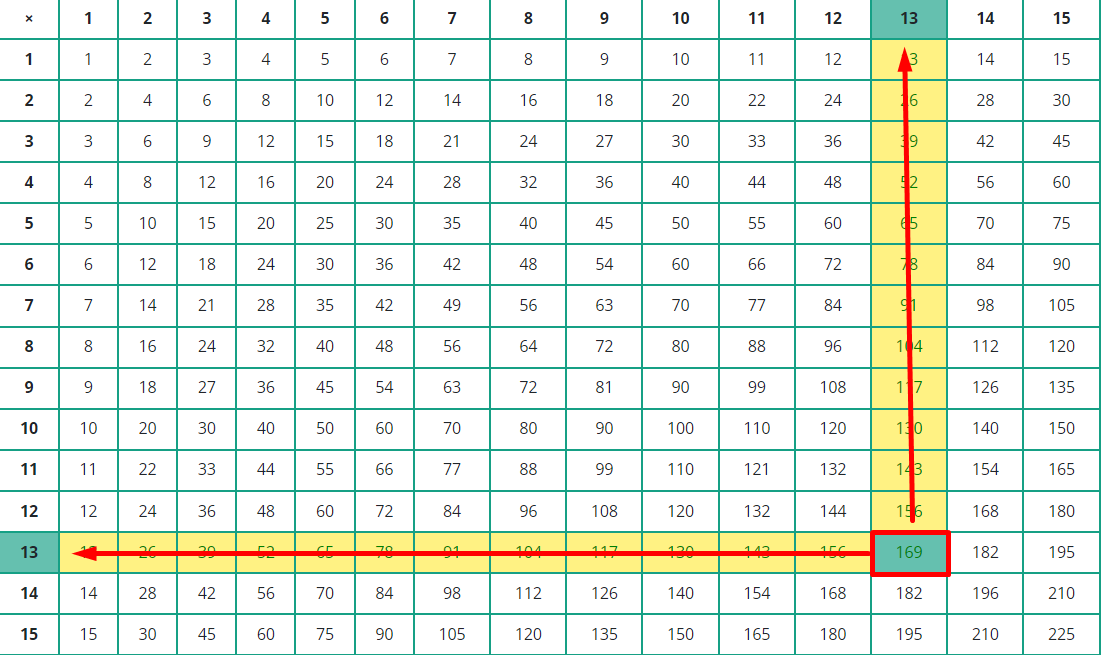Hence, 169 comes in multiplication table 13

How to see the product on the multiplication table?

Use a multiplication table chart to view the product of two numbers. If you want to view two numbers in a multiplication table, first of all, find the first number on the first row of the multiplication table chart and the second number on the first column, and then view the intersecting number that will be the product of two numbers.

For example, what is the `product of 12 and 14`?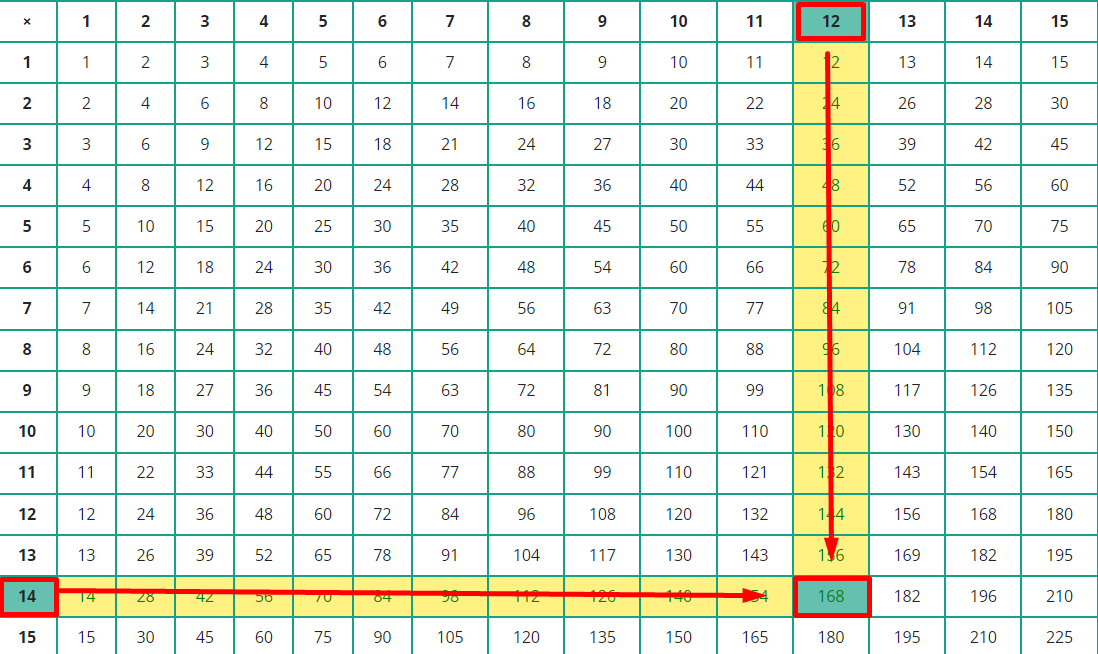Hence, the product of 12 and 14 is 168

How can learning tables 2 to 30 be helpful?

Learning times tables 2 to 30 are very helpful to solve and practice various multiplication facts. It is usually helpful in calculating math problems while performing multiplication, division, prime factorization, and math expressions.

You can easily learn times tables 2 to 30 by using the above times table chart. Tables 2 to 20 are present in the content. Here are tables 21 to 30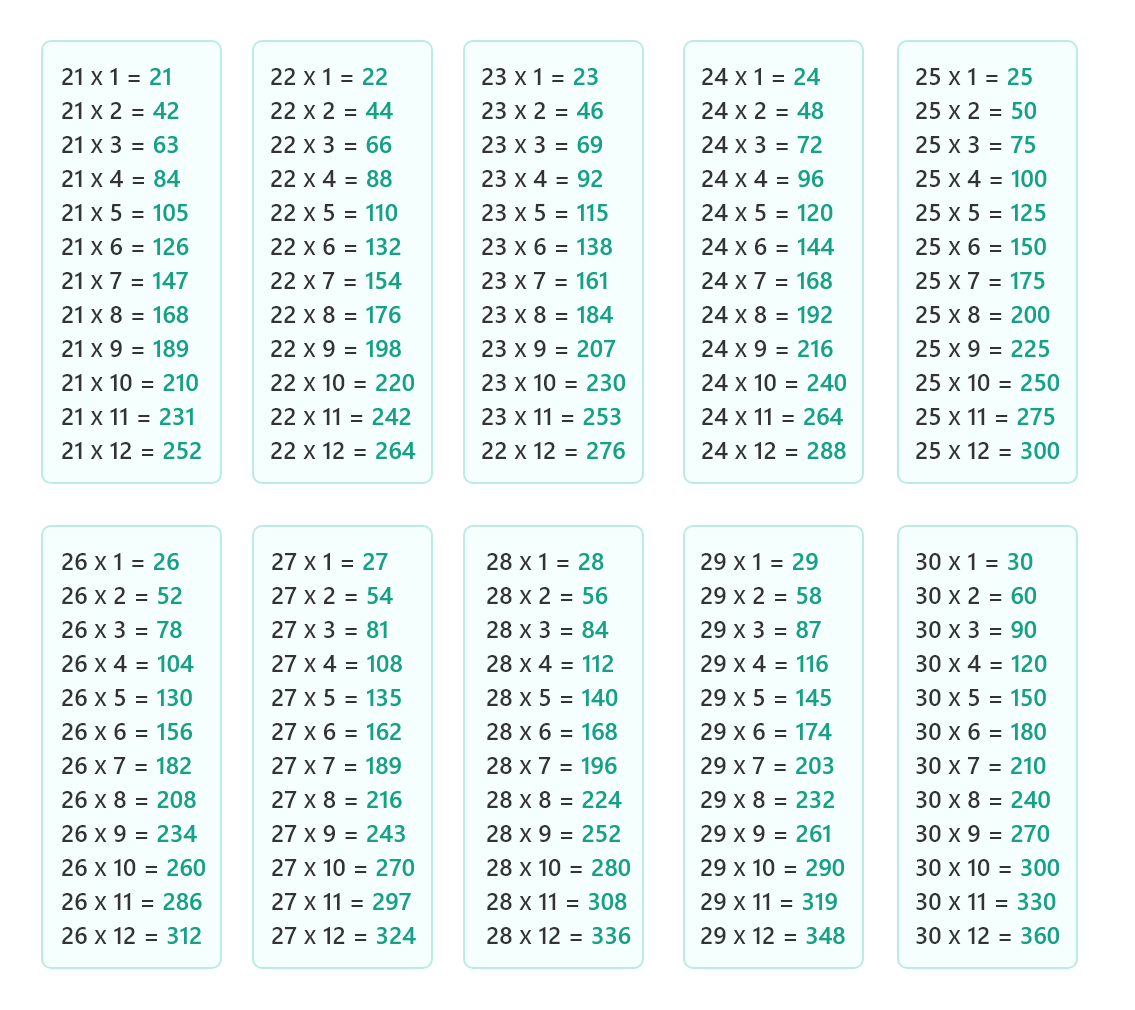### Math Tools